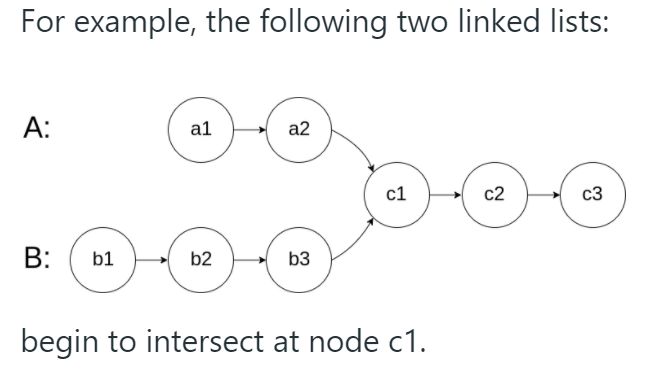# Leetcode — 160. Intersection of Two Linked Lists

Write a program to find the node at which the intersection of two singly linked lists begins.so the result should return the linked list that starts at c1

Notes:

• If the two linked lists have no intersection at all, return `null`.
• The linked lists must retain their original structure after the function returns.
• You may assume there are no cycles anywhere in the entire linked structure.
• Each value on each linked list is in the range `[1, 10^9]`.
• Your code should preferably run in O(n) time and use only O(1) memory.

# Thoughts:

This problem has been asked in a real interview, so it’s good to practice and know how we can solve it. The easiest way to solve this problem is to use a set that stores all the nodes in linked list A, and traverse all the nodes in list B to see if any node in B is exactly the same as the node in the set.

The 7ms will be like below, which wins 26%:

`public ListNode getIntersectionNode(ListNode headA, ListNode headB) {        Set<ListNode> set = new HashSet<>();        while(headA!=null)        {            set.add(headA);            headA = headA.next;        }        while(headB!=null)        {            if(set.contains(headB))                return headB;            headB = headB.next;        }        return null;    }`

Our previous code successfully runs in O(n) time complexity but it is required an O(n) space complexity. After you came up with this idea and present it to your interviewer, you might be asked to solve the problem using only O(1) space complexity.

# Code Logic:

Since the length of A and B are not guaranteed to be the same, we can do some tricks to let them be in the same length then compare them one by one.

For example, in the below graph, B is one node more than A. We will first move the head pointer of B forward one node, then compare A and B.

The reason why we can move the head pointer of the longer linked list forward is that we know these “redundant nodes” are impossible to be the “intersection of two linked lists”.

Hence, we can divide our code into 3 parts:

1. Find the length of A and B, to see if they have the same length.
2. Move the head pointer of the longer linked list till the two head pointers point to the same length linked list.
3. Compare the pointers one by one till the end of the linked list.

Below is the 0ms code that beats 100%:

`public ListNode getIntersectionNode(ListNode headA, ListNode headB) {        //step 1        ListNode temp = headA;        int lenA=0, lenB=0;        while(temp!=null)        {            temp=temp.next;            lenA++;        }        temp = headB;        while(temp!=null)        {            temp=temp.next;            lenB++;        }        //step 2        int diff=lenA-lenB;        while(diff>0) //A is longer        {            headA=headA.next;            diff--;        }        while(diff<0) //B is longer        {            headB=headB.next;            diff++;        }        //step 3        while(headA!=null)        {            if(headA==headB)                return headA;            headA=headA.next;            headB=headB.next;        }                return null;    }`

Time complexity O(n), space complexity O(1)

--

--